# Selina Concise Mathematics Class 6 ICSE Solutions Chapter 28 Polygons

## Selina Concise Mathematics Class 6 ICSE Solutions Chapter 28 Polygons

Selina Publishers Concise Mathematics Class 6 ICSE Solutions Chapter 28 Polygons

### Polygons Exercise 28A – Selina Concise Mathematics Class 6 ICSE Solutions

Question 1.
State, which of the following are polygons :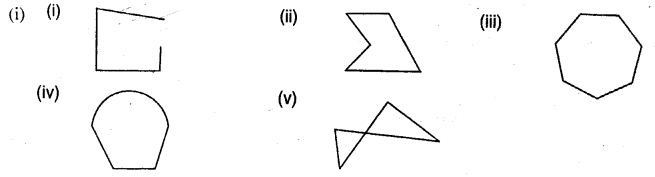Solution:Question 2.
Find the sum of interior angles of a polygon with :
(i) 9 sides
(ii) 13 sides
(iii) 16 sides
Solution:Question 3.
Find the number of sides of a polygon, if the sum of its interior angles is :
(i) 1440°
(ii) 1620°
Solution:Question 4.
Is it possible to have a polygon, whose sum of interior angles is 1030°.
Solution:Question 5.
(i) If all the angles of a hexagon arc equal, find the measure of each angle.
(ii) If all the angles of an octagon are equal, find the measure of each angle,
Solution: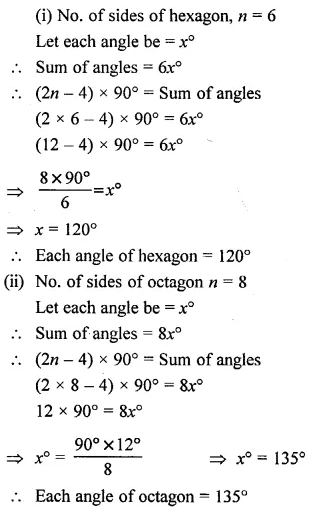Question 6.
One angle of a quadrilateral is 90° and all other angles are equal ; find each equal angle.
Solution:Question 7.
If angles of quadrilateral are in the ratio 4 : 5 : 3 : 6 ; find each angle of the quadrilateral.
Solution: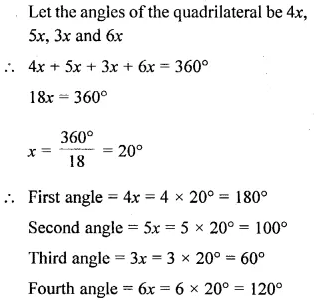Question 8.
If one angle of a pentagon is 120° and each of the remaining four angles is x°, find the magnitude of x.
Solution:Question 9.
The angles of a pentagon are in the ratio 5 : 4 : 5 : 7 : 6 ; find each angle of the pentagon.
Solution: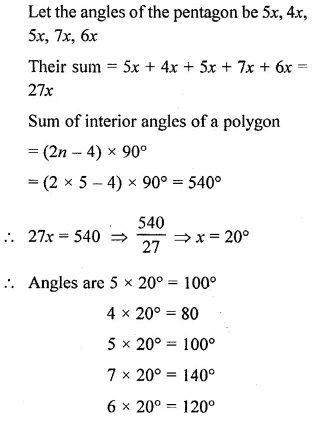Question 10.
Two angles of a hexagon are 90° and 110°. If the remaining four angles arc equal, find each equal angle.
Solution:### Polygons Exercise 28B – Selina Concise Mathematics Class 6 ICSE Solutions

Question 1.
Fill in the blanks :
In case of regular polygon, with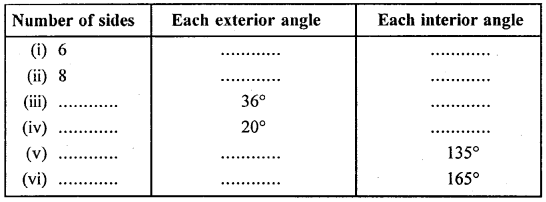Solution: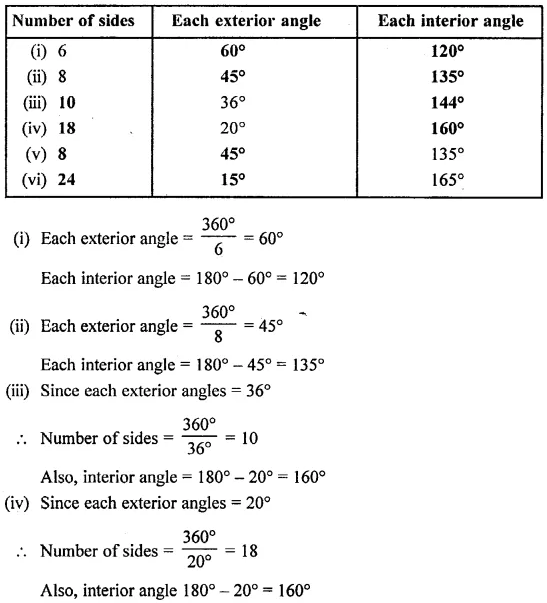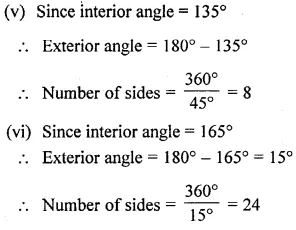Question 2.
Find the number of sides in a regular polygon, if its each interior angle is :
(i) 160°
(ii) 150°
Solution:Question 3.
Find number of sides in a regular polygon, if its each exterior angle is :
(i) 30°
(ii) 36°
Solution:Question 4.
Is it possible to have a regular polygon whose each interior angle is :
(i) 135°
(ii) 155°
Solution:Question 5.
Is it possible to have a regular polygon whose each exterior angle is :
(i) 100°
(ii) 36°
Solution:Question 6.
The ratio between the interior angle and the exterior angle of a regular polygon is 2 : 1. Find :
(i) each exterior angle of this polygon.
(ii) number of sides in the polygon.
Solution: